# How to validate if input in input field has BIC or Swift code only using express-validator ?

In HTML forms, we often required validation of different types. Validate existing email, validate password length, validate confirm password, validate to allow only integer inputs, these are some examples of validation. When working with a bank system, In a certain input field only a valid Bank identification code(BIC) is allowed. BIC is an 8 or 11 digit string. It represents a valid bank with its location uniquely. We can also validate these input fields to accept only valid BIC using express-validator middleware.

Condition to be a valid BIC:

• Bank Code: 4 letter code and only upper case alphabets are allowed.
• Country Code: 2 letter code and only upper case alphabets are allowed.
• Location Code: 2 letter code, either digits (0 to 9) or upper case alphabets are allowed.
• Branch Code: 3 letter optional code, either digits (0 to 9) or upper case alphabets are allowed.

Command to install express-validator:

`npm install express-validator`

Steps to use express-validator to implement the logic:

• Install express-validator middleware.
• Create a validator.js file to code all the validation logic.
• Validate input by validateInputField: check(input field name) and chain on the validation isBIC() with ‘ . ‘
• Use the validation name(validateInputField) in the routes as a middleware as an array of validations.
• Destructure ‘validationResult’ function from express-validator to use it to find any errors.
• If error occurs redirect to the same page passing the error information.
• If error list is empty, give access to the user for the subsequent request.

Note: Here we use local or custom database to implement the logic, the same steps can be followed to implement the logic in a regular database like MongoDB or MySql.

Example: This example illustrates how to validate a input field to accept only valid BIC.

Filename – index.js

 `const express = require(``'express'``) ` `const bodyParser = require(``'body-parser'``) ` `const {validationResult} = require(``'express-validator'``) ` `const repo = require(``'./repository'``) ` `const { validateBic } = require(``'./validator'``) ` `const formTemplet = require(``'./form'``) ` `const showTemplet = require(``'./show'``) ` ` `  `const app = express() ` `const port = process.env.PORT || 3000 ` ` `  `// The body-parser middleware to parse form data ` `app.use(bodyParser.urlencoded({extended : ``true``})) ` ` `  `// Get route to display HTML form ` `app.get(``'/'``, (req, res) => { ` `  ``res.send(formTemplet({})) ` `}) ` ` `  `// Post route to handle form submission logic and  ` `app.post( ` `  ``'/account'``, ` `  ``[validateBic], ` `  ``async (req, res) => { ` `    ``const errors = validationResult(req) ` `    ``if``(!errors.isEmpty()){ ` `      ``return` `res.send(formTemplet({errors})) ` `    ``} ` `    ``const {aNumber, bic} = req.body ` `    ``const account = await repo.getOneBy({ ` `      ``'accountNumber'``: aNumber, ` `      ``'bic'``: bic ` `    ``}) ` `    ``if``(account){ ` `      ``console.log(account) ` `      ``res.send(showTemplet(account)) ` `    ``}``else``{ ` `      ``res.send(``'Account Not Found'``) ` `    ``} ` `}) ` ` `  `// Server setup ` `app.listen(port, () => { ` `  ``console.log(`Server start on port \${port}`) ` `})`

Filename – repository.js: This file contains all the logic to create a local database and interact with it.

 `// Importing node.js file system module  ` `const fs = require(``'fs'``) ` ` `  `class Repository { ` `  ``constructor(filename) { ` `     `  `    ``// The filename where datas are going to store ` `    ``if``(!filename) { ` `      ``throw` `new` `Error(``'Filename is required to create a datastore!'``) ` `    ``} ` ` `  `    ``this``.filename = filename ` ` `  `    ``try` `{ ` `      ``fs.accessSync(``this``.filename) ` `    ``} ``catch``(err) { ` `      ``// If file not exist it is created with empty array ` `      ``fs.writeFileSync(``this``.filename, ``'[]'``) ` `    ``} ` `  ``} ` ` `  `  ``// Get all existing records ` `  ``async getAll(){ ` `    ``return` `JSON.parse( ` `      ``await fs.promises.readFile(``this``.filename, { ` `        ``encoding : ``'utf8'` `      ``}) ` `    ``) ` `  ``} ` ` `  `  ``// Find record by property ` `  ``async getOneBy(filters){ ` `    ``const records = await ``this``.getAll() ` `    ``for``(let record of records){ ` `      ``let found = ``true` `      ``for``(let key ``in` `filters){ ` `        ``if``(record[key] !== filters[key]){ ` `          ``found = ``false` `        ``} ` `      ``} ` `      ``if``(found) ``return` `record; ` `    ``} ` `  ``} ` ` `  `} ` ` `  `// The 'datastore.json' file created at runtime  ` `// and all the information provided via signup form ` `// store in this file in JSON format. ` `module.exports = ``new` `Repository(``'datastore.json'``)`

Filename – form.js: This file contains logic to show the form to fetch bank Information.

 `const getError = (errors, prop) => { ` `  ``try` `{ ` `    ``return` `errors.mapped()[prop].msg ` `  ``} ``catch` `(error) { ` `    ``return` `''` `  ``}  ` `} ` ` `  `module.exports = ({errors}) => { ` `  ``return` `` ` `    `` ` `    `` ` `      `` ` `        `` ` `        `` ` `      `` ` `      `` ` `        ``
` `          ``
` `            ``
` `              ``
` `                ``
` `                  ``
` `                    `` ` `                  ``
` `                  `` ` `                ``
` `                ``
` `                 ``
` `                  `` ` `                 ``
` `                 `` ` `                 ``

` `                   ``\${getError(errors, ``'bic'``)} ` `                 ``

` `                ``
` `                ``
` `                  `` ` `                ``
` `              `` ` `            ``
` `          ``
` `        ``
` `      `` ` `    ``    ` `  ``` ` `}`

Filename – show.js: This file contains logic to show the fetched bank information.

 `module.exports = (account) => { ` `  ``return` `` ` `    ``
` `      ``
` `        ``Account Name : ` `        ``\${account.accountName} ` `      ``
` `      ``
` `        ``Account Number : ` `        ``\${account.accountNumber} ` `      ``
` `      ``
` `        ``Account Type : ` `        ``\${account.accountType} ` `      ``
` `      ``
` `        ``BIC : ` `        ``\${account.bic} ` `      ``
` `      ``
` `        ``Bank Name : ` `        ``\${account.bankName} ` `      ``
` `      ``
` `        ``Bank Location : ` `        ``\${account.bankLocation} ` `      ``
` `    ``
` `  ``` ` `}`

Filename – validator.js: This file contain all the validation logic(Logic to validate a input field to accept only valid BIC).

 `const {check} = require(``'express-validator'``) ` `const repo = require(``'./repository'``) ` `module.exports = { ` `   `  `  ``validateBic : check(``'bic'``) ` ` `  `    ``// To delete leading and trialing space ` `    ``.trim() ` ` `  `    ``// Validate input field to accept only BIC ` `    ``.isBIC() ` `    ``.withMessage(``'Must be a valid Bank identification code'``) ` `}`

Filename – package.json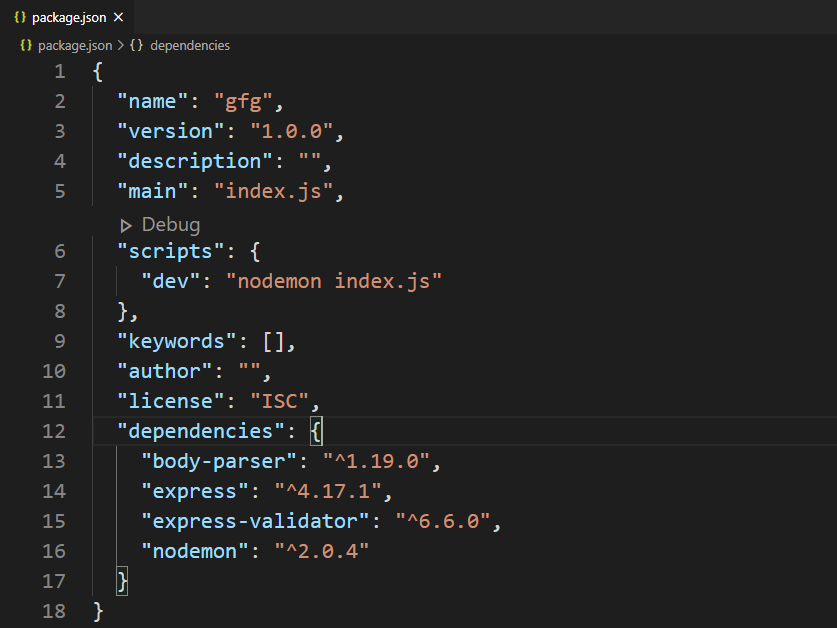package.json file

Database: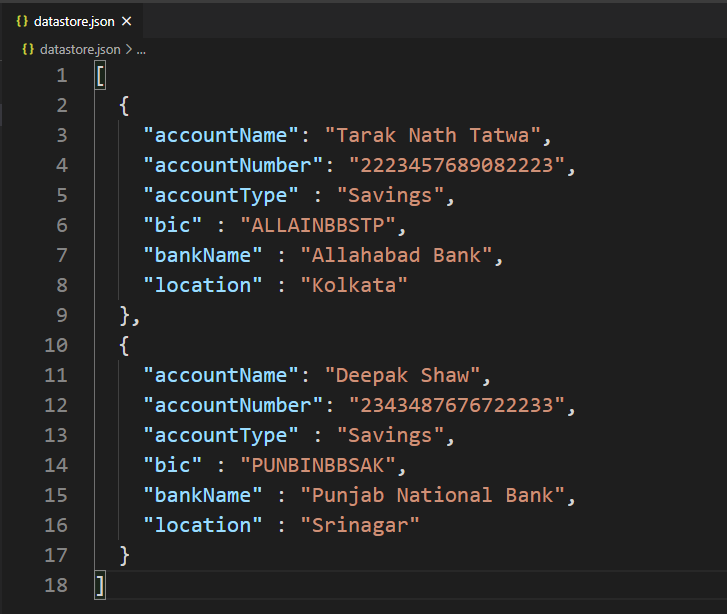Database

Output: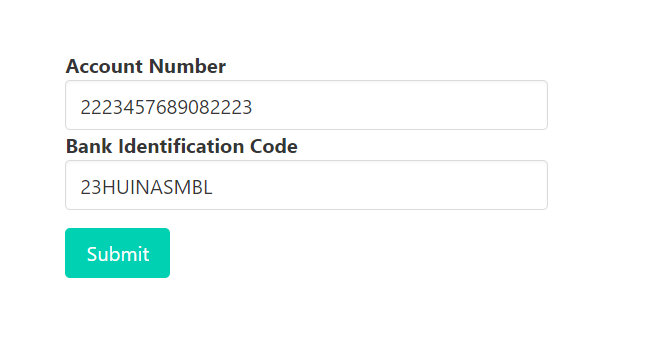Invalid first two digit(Only Alphabets allowed)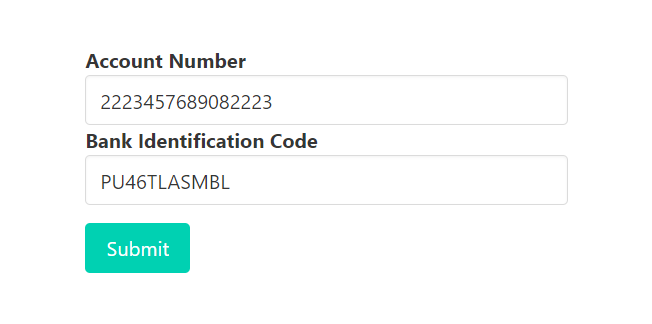Invalid 3rd and 4th digit(Only Alphabets allowed)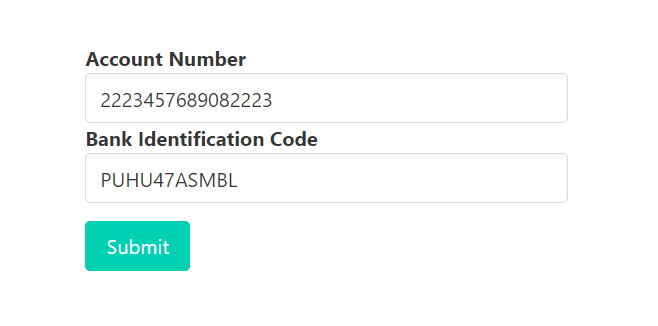Invalid 5th and 6th digit(Only Alphabets allowed)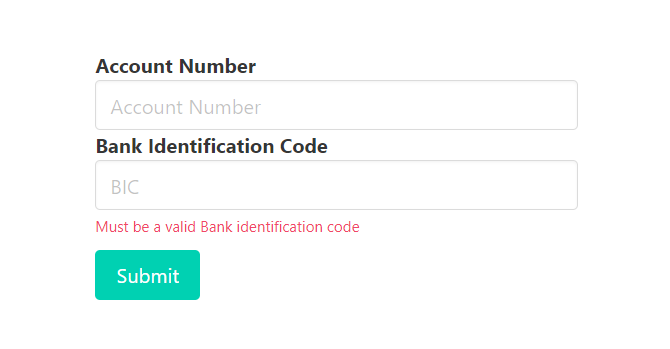Response when submit invalid BIC(In first three cases)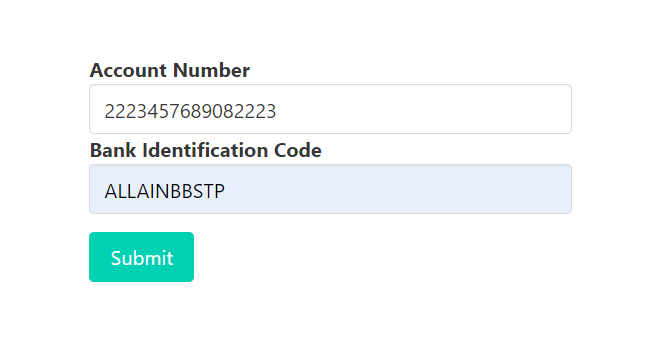valid BIC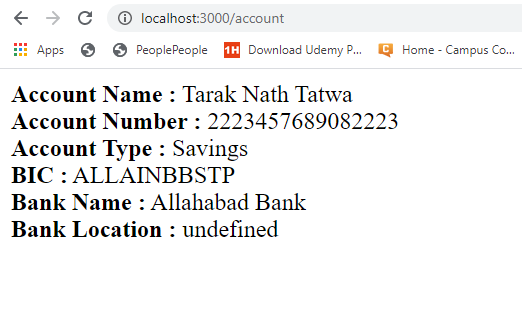Response when submit valid BIC

Note: We have used some Bulma classes(CSS framework) in the form.js file to design the content.

My Personal Notes arrow_drop_upCheck out this Author's contributed articles.

If you like GeeksforGeeks and would like to contribute, you can also write an article using contribute.geeksforgeeks.org or mail your article to contribute@geeksforgeeks.org. See your article appearing on the GeeksforGeeks main page and help other Geeks.

Please Improve this article if you find anything incorrect by clicking on the "Improve Article" button below.

Article Tags :

Be the First to upvote.

Please write to us at contribute@geeksforgeeks.org to report any issue with the above content.